Dividing Whole Numbers: Properties of Division

Learning Outcomes

• Divide whole numbers and check the answer using multiplication
• Identify and apply the division properties of one
• Identify and apply the division properties of zero
• Use the long division algorithm to divide multiple-digit numbers
• Identify the divisor, dividend, and remainder in a division problem

Divide Whole Numbers

We said that addition and subtraction are inverse operations because one undoes the other. Similarly, division is the inverse operation of multiplication. We know $12\div 4=3$ because $3\cdot 4=12$. Knowing all the multiplication number facts is very important when doing division.

We check our answer to division by multiplying the quotient by the divisor to determine if it equals the dividend. We know $24\div 8=3$ is correct because $3\cdot 8=24$.

example

Divide. Then check by multiplying.

1. $42\div 6$
2. $\frac{72}{9}$
3. $7\overline{)63}$

Solution:

 1. $42\div 6$ Divide $42$ by $6$. $7$ Check by multiplying. $7\cdot 6$ $42\quad\checkmark$
 2. $\frac{72}{9}$ Divide $72$ by $9$. $8$ Check by multiplying. $8\cdot 9$ $72\quad\checkmark$
 3. $7\overline{)63}$ Divide $63$ by $7$. $9$ Check by multiplying. $9\cdot 7$ $63\quad\checkmark$

try it

What is the quotient when you divide a number by itself?

$\frac{15}{15}=1$

because $1\cdot 15=15$

Dividing any number $\text{(except 0)}$ by itself produces a quotient of $1$. Also, any number divided by $1$ produces a quotient of the number. These two ideas are stated in the Division Properties of One.

Division Properties of One

 Any number (except 0) divided by itself is one. $a\div a=1$ Any number divided by one is the same number. $a\div 1=a$

example

Divide. Then check by multiplying:

1. $11\div 11$
2. $\frac{19}{1}$
3. $1\overline{)7}$

try it

Suppose we have $\text{\0}$, and want to divide it among $3$ people. How much would each person get? Each person would get $\text{\0}$. Zero divided by any number is $0$.

Now suppose that we want to divide $\text{\10}$ by $0$. That means we would want to find a number that we multiply by $0$ to get $10$. This cannot happen because $0$ times any number is $0$. Division by zero is said to be undefined.

These two ideas make up the Division Properties of Zero.

Division Properties of Zero

 Zero divided by any number is $0$. $0\div a=0$ Dividing a number by zero is undefined. $a\div 0$ undefined

Another way to explain why division by zero is undefined is to remember that division is really repeated subtraction. How many times can we take away $0$ from $10?$ Because subtracting $0$ will never change the total, we will never get an answer. So we cannot divide a number by $0$.

example

Divide. Check by multiplying:

1. $0\div 3$
2. $\frac{10}{0}$

try it

When the divisor or the dividend has more than one digit, it is usually easier to use the $4\overline{)12}$ notation. This process is called long division. Let’s work through the process by dividing $78$ by $3$.

 Divide the first digit of the dividend, $7$, by the divisor, $3$. The divisor $3$ can go into $7$ two times since $2\times 3=6$ . Write the $2$ above the $7$ in the quotient.Multiply the $2$ in the quotient by $2$ and write the product, $6$, under the$7$.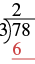Subtract that product from the first digit in the dividend. Subtract $7 - 6$ . Write the difference, 1, under the first digit in the dividend.Bring down the next digit of the dividend. Bring down the $8$.Divide $18$ by the divisor, $3$. The divisor $3$ goes into $18$ six times.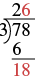Write $6$ in the quotient above the $8$. Multiply the $6$ in the quotient by the divisor and write the product, $18$, under the dividend. Subtract $18$ from $18$.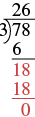We would repeat the process until there are no more digits in the dividend to bring down. In this problem, there are no more digits to bring down, so the division is finished.

$\text{So }78\div 3=26$.

Check by multiplying the quotient times the divisor to get the dividend. Multiply $26\times 3$ to make sure that product equals the dividend, $78$.

$\begin{array}{c}\hfill \stackrel{1}{2}6\\ \hfill \underset{\text{___}}{\times 3}\\ \hfill 78 \end{array}$

It does, so our answer is correct. $\checkmark$

Divide whole numbers

1. Divide the first digit of the dividend by the divisor.If the divisor is larger than the first digit of the dividend, divide the first two digits of the dividend by the divisor, and so on.
2. Write the quotient above the dividend.
3. Multiply the quotient by the divisor and write the product under the dividend.
4. Subtract that product from the dividend.
5. Bring down the next digit of the dividend.
6. Repeat from Step 1 until there are no more digits in the dividend to bring down.
7. Check by multiplying the quotient times the divisor.

In the video below we show another example of using long division.

example

Divide and then check by multiplying:

$2,596\div 4$

example

Divide and then check by multiplying:

$4,506\div 6$

example

Divide and then check by multiplying:

$7,263\div 9$

try it

Watch this video for another example of how to use long division to divide a four digit whole number by a two digit whole number.

So far all the division problems have worked out evenly. For example, if we had $24$ cookies and wanted to make bags of $8$ cookies, we would have $3$ bags. But what if there were $28$ cookies and we wanted to make bags of $8?$ Start with the $28$ cookies.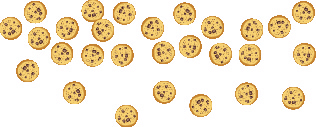Try to put the cookies in groups of eight.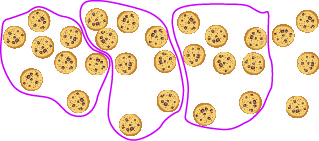There are $3$ groups of eight cookies, and $4$ cookies left over. We call the $4$ cookies that are left over the remainder and show it by writing R4 next to the $3$. (The R stands for remainder.)

To check this division we multiply $3$ times $8$ to get $24$, and then add the remainder of $4$.

$\begin{array}{c}\hfill 3\\ \hfill \underset{\text{___}}{\times 8}\\ \hfill 24\\ \hfill \underset{\text{___}}{+4}\\ \hfill 28\end{array}$

example

Divide and then check by multiplying:

$1,439\div 4$

example

Divide and then check by multiplying:

$1,461\div 13$

try it

Sometimes it might not be obvious how many times the divisor goes into digits of the dividend. We will have to guess and check numbers to find the greatest number that goes into the digits without exceeding them.

example

Divide and check by multiplying:

$74,521\div 241$

try it

Watch the video below for another example of how to use long division to divide whole numbers when there is a remainder.